Gravity

What is gravity?

Gravity is an attractive force, one that attracts all of the matter in the Universe towards all of the other bits of matter in the Universe. On the size scale of moons, planets, stars, and galaxies, it is an extremely important force, and governs much of the behavior of these objects. Gravity keeps our feet firmly on the ground, keeps the Moon in orbit around the Earth, keeps the Earth in orbit around the Sun, keeps the Sun in orbit around the center of our Milky Way galaxy, keeps the Milky Way and Andromeda galaxies orbiting their common center of mass, and so on, and so on ... for matter, gravity really matters!

When dealing with the force of gravity between two objects, there are only two things that are important – mass, and distance. The force of gravity depends directly upon the masses of the two objects, and inversely on the square of the distance between them. This means that the force of gravity increases with mass, but decreases with increasing distance between objects.

We are drawn towards the most massive objects, and towards the closest objects. Even though the Sun is far more massive than the Earth, the Earth's close proximity insures that our feet stay planted on terra firma rather than zooming off toward the Sun. A space craft docked on Earth acts the same way; but if we fire it off toward the Moon, there will come a time at which the Moon's weak gravitation attraction outweighs that of the more distant Earth, and the space craft will begin to drift toward the lunar surface.

By how much does gravitational attraction increase with increasing mass (M1 and M2) and by how much does it decrease with increasing distance (R)? For a gravitational force, F,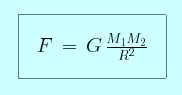where G is a constant factor (the gravitational constant), which does not vary.

Since the distance term is squared (the exponent is a two), the force of gravity falls by a factor of four when the distance is doubled (as two squared is four), and by a factor of nine when it is tripled (as three squared is nine).

However, the exponent on the mass terms is one. This means that if one of the objects suddenly became ten times more massive, the gravitational attraction between the two objects would grow by ten times as well.

What is the difference between force and acceleration?

You may have noticed that the gravitational force equation is symmetric for our two objects – does this mean that the gravitational force that you exert on the Earth is as strong as that exerted on you by the Earth? Yes!

This may seem puzzling at first, so let's take care to distinguish between force, F, and acceleration, a. Your gravitational acceleration is the rate at which your speed increases as you are drawn toward another object (how quickly you become attracted to it). Your gravitational force is the product of your acceleration and your mass, m.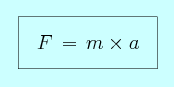Let's consider the gravitational force between you and the Earth. As above, your mass is m and your acceleration is a. The Earth's mass and acceleration are M and A, and the distance between you and the Earth is R. (You can think of R as the Earth's radius.)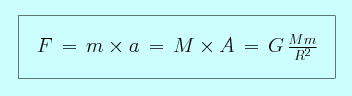It is clear that the force that you exert on the Earth is a large as the force that the Earth exerts on you. However, how does your acceleration toward the center of the Earth compare to the Earth's acceleration toward you?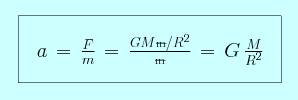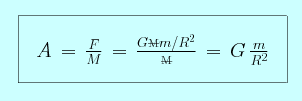Because your mass is much less than that of the Earth (m << M), your experience a much greater acceleration than the Earth does (a >> A)! This is why if you toss a ball into the air, it is pulled back to Earth rather than pulling the whole Earth to it.

In a certain sense, the force tells you how hard you are being pulled, and the acceleration tells you how much you move in response. The more massive an object is, the harder one must pull to move it. (As anyone who has ever tried to help a friend arrange, and re-arrange, their living room furniture set knows well.)

On the surface of the Earth, the gravitational force is what we call your weight, and the gravitational acceleration is equivalent to the surface gravity, g, equal to 980 centimeters per second squared.